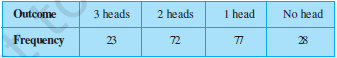#### Q4. Three coins are tossed simultaneously 200 times with the following frequencies of different outcomes:If the three coins are simultaneously tossed again, compute the probability of 2 heads coming up.

From the above question, the data of our interest is:

Total no. of times the coins(i.e. 3 coins simultaneously) are tossed =200

No. of times the result comes out  with 2 heads=72

The empirical (or experimental) probability P(E) of this event is given by :=72/200

= 9/25

Ans: 9/25

#### Safeer PP